JAVA程序实例之动手实现一个 LRU cache白羽 2018-07-09 来源 ：网络 阅读 151 评论 0· 实现一个 LRU 缓存，当缓存数据达到 N 之后需要淘汰掉最近最少使用的数据。

· N 小时之内没有被访问的数据也需要淘汰掉。

```public class LRUAbstractMap extends java.util.AbstractMap {

private final static Logger LOGGER = LoggerFactory.getLogger(LRUAbstractMap.class);

/**
* 检查是否超期线程
*/
private ExecutorService checkTimePool ;

/**
* map 最大size
*/
private final static int MAX_SIZE = 1024 ;

private final static ArrayBlockingQueue<Node> QUEUE = new ArrayBlockingQueue<>(MAX_SIZE) ;

/**
* 默认大小
*/
private final static int DEFAULT_ARRAY_SIZE =1024 ;

/**
* 数组长度
*/
private int arraySize ;

/**
* 数组
*/
private Object[] arrays ;

/**
* 判断是否停止 flag
*/
private volatile boolean flag = true ;

/**
* 超时时间
*/
private final static Long EXPIRE_TIME = 60 * 60 * 1000L ;

/**
* 整个 Map 的大小
*/
private volatile AtomicInteger size  ;

public LRUAbstractMap() {

arraySize = DEFAULT_ARRAY_SIZE;
arrays = new Object[arraySize] ;

//开启一个线程检查最先放入队列的值是否超期
executeCheckTime();
}

/**
* 开启一个线程检查最先放入队列的值是否超期 设置为守护线程
*/
private void executeCheckTime() {
.setDaemon(true)
.build();
checkTimePool = new ThreadPoolExecutor(1, 1, 0L, TimeUnit.MILLISECONDS,

}

@Override
public Set<Entry> entrySet() {
return super.keySet();
}

@Override
public Object put(Object key, Object value) {
int hash = hash(key);
int index = hash % arraySize ;
Node currentNode = (Node) arrays[index] ;

if (currentNode == null){
arrays[index] = new Node(null,null, key, value);

//写入队列
QUEUE.offer((Node) arrays[index]) ;

sizeUp();
}else {
Node cNode = currentNode ;
Node nNode = cNode ;

//存在就覆盖
if (nNode.key == key){
cNode.val = value ;
}

while (nNode.next != null){
//key 存在 就覆盖 简单判断
if (nNode.key == key){
nNode.val = value ;
break ;
}else {
//不存在就新增链表
sizeUp();
Node node = new Node(nNode,null,key,value) ;

//写入队列
QUEUE.offer(currentNode) ;

cNode.next = node ;
}

nNode = nNode.next ;
}

}

return null ;
}

@Override
public Object get(Object key) {

int hash = hash(key) ;
int index = hash % arraySize ;
Node currentNode = (Node) arrays[index] ;

if (currentNode == null){
return null ;
}
if (currentNode.next == null){

//更新时间
currentNode.setUpdateTime(System.currentTimeMillis());

//没有冲突
return currentNode ;

}

Node nNode = currentNode ;
while (nNode.next != null){

if (nNode.key == key){

//更新时间
currentNode.setUpdateTime(System.currentTimeMillis());

return nNode ;
}

nNode = nNode.next ;
}

return super.get(key);
}

@Override
public Object remove(Object key) {

int hash = hash(key) ;
int index = hash % arraySize ;
Node currentNode = (Node) arrays[index] ;

if (currentNode == null){
return null ;
}

if (currentNode.key == key){
sizeDown();
arrays[index] = null ;

//移除队列
QUEUE.poll();
return currentNode ;
}

Node nNode = currentNode ;
while (nNode.next != null){

if (nNode.key == key){
sizeDown();
//在链表中找到了 把上一个节点的 next 指向当前节点的下一个节点
nNode.pre.next = nNode.next ;
nNode = null ;

//移除队列
QUEUE.poll();

return nNode;
}

nNode = nNode.next ;
}

return super.remove(key);
}

/**
* 增加size
*/
private void sizeUp(){

//在put值时候认为里边已经有数据了
flag = true ;

if (size == null){
size = new AtomicInteger() ;
}
int size = this.size.incrementAndGet();
if (size >= MAX_SIZE) {
//找到队列头的数据
Node node = QUEUE.poll() ;
if (node == null){
throw new RuntimeException("data error") ;
}

//移除该 key
Object key = node.key ;
remove(key) ;
lruCallback() ;
}

}

/**
* 数量减小
*/
private void sizeDown(){

if (QUEUE.size() == 0){
flag = false ;
}

this.size.decrementAndGet() ;
}

@Override
public int size() {
return size.get() ;
}

/**
* 链表
*/
private class Node{
private Node next ;
private Node pre ;
private Object key ;
private Object val ;
private Long updateTime ;

public Node(Node pre,Node next, Object key, Object val) {
this.pre = pre ;
this.next = next;
this.key = key;
this.val = val;
this.updateTime = System.currentTimeMillis() ;
}

public void setUpdateTime(Long updateTime) {
this.updateTime = updateTime;
}

public Long getUpdateTime() {
return updateTime;
}

@Override
public String toString() {
return "Node{" +
"key=" + key +
", val=" + val +
'}';
}
}

/**
* copy HashMap 的 hash 实现
* @param key
* @return
*/
public int hash(Object key) {
int h;
return (key == null) ? 0 : (h = key.hashCode()) ^ (h >>> 16);
}

private void lruCallback(){
LOGGER.debug("lruCallback");
}

private class CheckTimeThread implements Runnable{

@Override
public void run() {
while (flag){
try {
Node node = QUEUE.poll();
if (node == null){
continue ;
}
Long updateTime = node.getUpdateTime() ;

if ((updateTime - System.currentTimeMillis()) >= EXPIRE_TIME){
remove(node.key) ;
}
} catch (Exception e) {
LOGGER.error("InterruptedException");
}
}
}
}

}

https://github.com/crossoverJie/Java-Interview/blob/master/src/main/java/com/crossoverjie/actual/LRUAbstractMap.java

· 采用了与 HashMap 一样的保存数据方式，只是自己手动实现了一个简易版。
· 内部采用了一个队列来保存每次写入的数据。
· 写入的时候判断缓存是否大于了阈值 N，如果满足则根据队列的 FIFO 特性将队列头的数据删除。因为队列头的数据肯定是最先放进去的。
· 再开启了一个守护线程用于判断最先放进去的数据是否超期（因为就算超期也是最先放进去的数据最有可能满足超期条件。）
· 设置为守护线程可以更好的表明其目的（最坏的情况下，如果是一个用户线程最终有可能导致程序不能正常退出，因为该线程一直在运行，守护线程则不会有这个情况。）

· 要记录最近最少使用，那至少需要一个有序的集合来保证写入的顺序。
· 在使用了数据之后能够更新它的顺序。

1. 每次写入数据时将数据放入链表头结点。
2. 使用数据时候将数据移动到头结点。
3. 缓存数量超过阈值时移除链表尾部数据。

public class LRUMap<K, V> {
private final Map<K, V> cacheMap = new HashMap<>();

/**
* 最大缓存大小
*/
private int cacheSize;

/**
* 节点大小
*/
private int nodeCount;

/**
* 头结点
*/
private Node<K, V> header;

/**
* 尾结点
*/
private Node<K, V> tailer;

public LRUMap(int cacheSize) {
this.cacheSize = cacheSize;
//头结点的下一个结点为空
header = new Node<>();

//尾结点的上一个结点为空
tailer = new Node<>();
tailer.tail = null;

//双向链表 头结点的上结点指向尾结点

//尾结点的下结点指向头结点

}

public void put(K key, V value) {
cacheMap.put(key, value);

//双向链表中添加结点
}

public V get(K key){

Node<K, V> node = getNode(key);

//移动到头结点

return cacheMap.get(key);
}

private void moveToHead(Node<K,V> node){

//如果是最后的一个节点
if (node.tail == null){
node.next.tail = null ;
tailer = node.next ;
nodeCount -- ;
}

//如果是本来就是头节点 不作处理
if (node.next == null){
return ;
}

//如果处于中间节点
if (node.tail != null && node.next != null){
//它的上一节点指向它的下一节点 也就删除当前节点
node.tail.next = node.next ;
nodeCount -- ;
}

//最后在头部增加当前节点
//注意这里需要重新 new 一个对象，不然原本的node 还有着下面的引用，会造成内存溢出。
node = new Node<>(node.getKey(),node.getValue()) ;

}

/**
* 链表查询 效率较低
* @param key
* @return
*/
private Node<K,V> getNode(K key){
Node<K,V> node = tailer ;
while (node != null){

if (node.getKey().equals(key)){
return node ;
}

node = node.next ;
}

return null ;
}

/**
* 写入头结点
* @param key
* @param value
*/
private void addNode(K key, V value) {

Node<K, V> node = new Node<>(key, value);

//容量满了删除最后一个
if (cacheSize == nodeCount) {
//删除尾结点
delTail();
}

//写入头结点

}

/**
* 添加头结点
*
* @param node
*/

//写入头结点
nodeCount++;

//如果写入的数据大于2个 就将初始化的头尾结点删除
if (nodeCount == 2) {
tailer.next.next.tail = null;
tailer = tailer.next.next;
}

}

private void delTail() {
//把尾结点从缓存中删除
cacheMap.remove(tailer.getKey());

//删除尾结点
tailer.next.tail = null;
tailer = tailer.next;

nodeCount--;

}

private class Node<K, V> {
private K key;
private V value;
Node<K, V> tail;
Node<K, V> next;

public Node(K key, V value) {
this.key = key;
this.value = value;
}

public Node() {
}

public K getKey() {
return key;
}

public void setKey(K key) {
this.key = key;
}

public V getValue() {
return value;
}

public void setValue(V value) {
this.value = value;
}

}

@Override
public String toString() {
StringBuilder sb = new StringBuilder() ;
Node<K,V> node = tailer ;
while (node != null){
sb.append(node.getKey()).append(":")
.append(node.getValue())
.append("-->") ;

node = node.next ;
}

return sb.toString();
}
}

@Test
public void put() throws Exception {
LRUMap<String,Integer> lruMap = new LRUMap(3) ;
lruMap.put("1",1) ;
lruMap.put("2",2) ;
lruMap.put("3",3) ;

System.out.println(lruMap.toString());

lruMap.put("4",4) ;
System.out.println(lruMap.toString());

lruMap.put("5",5) ;
System.out.println(lruMap.toString());
}
//输出：1:1-->2:2-->3:3-->2:2-->3:3-->4:4-->3:3-->4:4-->5:5-->

@Test
public void get() throws Exception {
LRUMap<String,Integer> lruMap = new LRUMap(3) ;
lruMap.put("1",1) ;
lruMap.put("2",2) ;
lruMap.put("3",3) ;

System.out.println(lruMap.toString());
System.out.println("==============");

Integer integer = lruMap.get("1");
System.out.println(integer);
System.out.println("==============");
System.out.println(lruMap.toString());
}
//输出1:1-->2:2-->3:3-->
==============1
==============2:2-->3:3-->1:1-->

· 数据是直接利用 HashMap 来存放的。
· 内部使用了一个双向链表来存放数据，所以有一个头结点 header，以及尾结点 tailer。
· 每次写入头结点，删除尾结点时都是依赖于 header tailer，如果看着比较懵建议自己实现一个链表熟悉下，或结合下文的对象关系图一起理解。
· 使用数据移动到链表头时，第一步是需要在双向链表中找到该节点。这里就体现出链表的问题了。查找效率很低，最差需要 O(N)。之后依赖于当前节点进行移动。
· 在写入头结点时有判断链表大小等于 2 时需要删除初始化的头尾结点。这是因为初始化时候生成了两个双向节点，没有数据只是为了形成一个数据结构。当真实数据进来之后需要删除以方便后续的操作（这点可以继续优化）。
· 以上的所有操作都是线程不安全的，需要使用者自行控制。

1

LRUMap<String,Integer> lruMap = new LRUMap(3) ;
lruMap.put("1",1) ;
2
lruMap.put("2",2) ;
3
lruMap.put("3",3) ;
4
lruMap.put("4",4) ;
5

Integer integer = lruMap.get("2");
6

public class LRULinkedMap<K,V> {

/**
* 最大缓存大小
*/
private int cacheSize;

private LinkedHashMap<K,V> cacheMap ;

public LRULinkedMap(int cacheSize) {
this.cacheSize = cacheSize;

cacheMap = new LinkedHashMap(16,0.75F,true){
@Override
protected boolean removeEldestEntry(Map.Entry eldest) {
if (cacheSize + 1 == cacheMap.size()){
return true ;
}else {
return false ;
}
}
};
}

public void put(K key,V value){
cacheMap.put(key,value) ;
}

public V get(K key){
return cacheMap.get(key) ;
}

public Collection<Map.Entry<K, V>> getAll() {
return new ArrayList<Map.Entry<K, V>>(cacheMap.entrySet());
}
}

@Test
public void put() throws Exception {
map.put("1",1);
map.put("2",2);
map.put("3",3);

for (Map.Entry<String, Integer> e : map.getAll()){
System.out.print(e.getKey() + " : " + e.getValue() + "\t");
}

System.out.println("");
map.put("4",4);
for (Map.Entry<String, Integer> e : map.getAll()){
System.out.print(e.getKey() + " : " + e.getValue() + "\t");
}
}
//输出1 : 1    2 : 2    3 : 3    2 : 2    3 : 3    4 : 4

@Test
public void get() throws Exception {
map.put("1",1);
map.put("2",2);
map.put("3",3);
map.put("4",4);

for (Map.Entry<String, Integer> e : map.getAll()){
System.out.print(e.getKey() + " : " + e.getValue() + "\t");
}

System.out.println("");
map.get("1") ;
for (Map.Entry<String, Integer> e : map.getAll()){
System.out.print(e.getKey() + " : " + e.getValue() + "\t");
}
}

}
//输出1 : 1    2 : 2    3 : 3    4 : 4    2 : 2    3 : 3    4 : 4    1 : 1

protected boolean removeEldestEntry(Map.Entry<K,V> eldest) {
return false;
}```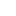喜欢 | 2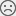不喜欢 | 0

JAVA工程师交流群 +加入

WEB/H5前端交流群 +加入•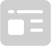资料索取
•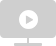答疑解惑
•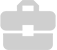技术交流
•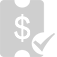职业测评
•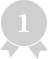面试技巧
•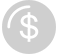高薪秘笈
JAVA30天热搜词 更多>>
X沪公网安备 31011502005948号    ICP许可  沪B2-20190160